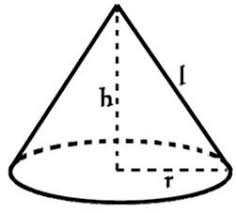## How to Calculate and Solve for the Area, Radius, Diameter and Slant Height of a Cone | The Calculator EncyclopediaThe image above is a cone.

To compute the area of a cone, two essential parameters is needed and this parameters are the radius of the cone (r) and the slant height of the cone (h).

The formula for calculating the area of a cone:

A = πrl + πr²

Where;

A = Area of the Cone
r = Radius of the Cone

Let’s solve an example:
Find the area of a cone when the radius of the cone is 9 cm and the slant height of the cone is 12 cm.

This implies that;
r = Radius of the cone = 9 cm
l = Slant height of the cone = 12 cm

A = πrl + πr²
A = 3.142 x 9 x 12 + 3.142 x 9²
A = 339.336 + 254.502
A =  593.83

Therefore, the area of the cone is 593.83 cm².

Calculating the Area of a cone using Diameter and Slant height of the cone.

A = πdl / 2 + πd2 / 4

Where;

d = Diameter of the Cone
l = Slant height of the Cone

Let’s solve an example:
Find the area of a cone when the diameter of the cone is 18 cm and the slant height of the cone is 22 cm?

This implies that;
d = diameter of the cone = 18 cm
l = Slant height of the cone = 22 cm

A = πdl / 2 + πd2 / 4
A = 3.142 x 18 x 22 / 2 + 3.142 (18)2 / 4
A = 1244.232 / 2 + 1018.008 / 4
A = 622.116 + 254.502
A = 876.6

Therefore, the area of the cone with diameter is 876.6 cm2.

Calculating the Slant height of a cone using Radius of the cone and Area of the cone.

l = A – πr2 / πr

Where;

A = Area of the Cone
r = Radius of the Cone

Let’s solve an example:
Find the slant height of a cone when the radius of the cone is 8 cm and the area of the cone is 220 cm2.

This implies that;
A = Area of the cone = 220 cm2
r = Radius of the cone = 8 cm

l = A – πr2 / πr
l = 220 – 3.142 x 82 / 3.142 x 8
l = 220 – 3.142 x 64 / 25.136
l = 220 – 201.088 / 25.136
l = 18.91 / 25.136
l = 0.75

Therefore, the slant height of the cone with radius is 0.75 cm.